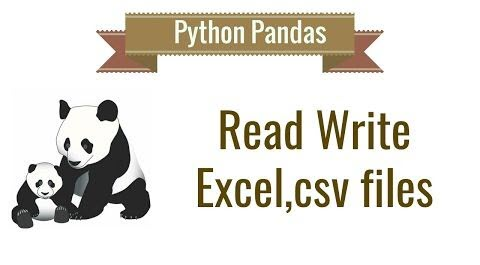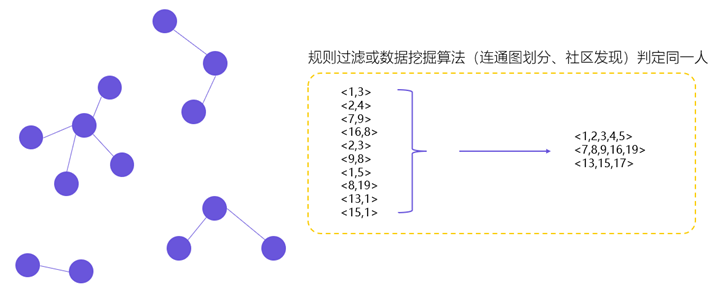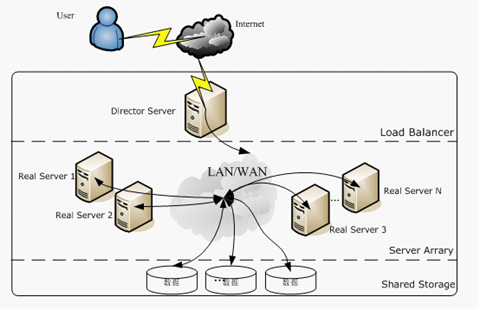Pandas读取与导出Excel、CSV文件加载Excel文件

pandas.read_excel(io, sheet_name=0, header=0, names=None, index_col=None, usecols=None, squeeze=False, dtype=None, engine=None, converters=None, true_values=None, false_values=None, skiprows=None, nrows=None, na_values=None, parse_dates=False, date_parser=None, thousands=None, comment=None, skipfooter=0, convert_float=True, **kwds)

• io：excel文件，可以是文件路径、文件网址、file-like对象、xlrd workbook
• sheetname：返回指定的sheet，参数可以是字符串（sheet名）、整型（sheet索引）、list（元素为字符串和整型，返回字典{‘key’:’sheet’}）、none（返回字典，全部sheet）
• names：返回指定name的列，参数为array-like对象。
• index_col：设定索引的列，参数可以是int、list of ints
• usecol：设定需要解析的列，默认为None，代表解析素有，如果直传一个int，代表解析到最后的那个列，如果传的是list则返回的是限定的列，比如：“A:E”或“A,C,E:F”
• squeeze：如果解析的数据只包含一列数据，则返回一个Series，默认返回为DataFrame
• dtype：可以制定每列的类型，示例：{‘a’: np.float64, ‘b’: np.int32}
• engine：如果 io 不是缓冲区或路径，则必须设置 io。 可接受的值是 None 或 xlrd
• converters：自定形式，设定对应的列要用的转换函数。
• true_values：设定安歇为True值，不常用
• false_values：设定哪些为False值，不常用
• shiprows：需要跳过的行，list-like类型
• nrows：要分析的行数
• na_values：N/A值列表
• parse_dates：传入的是list，将指定的类解析为date格式
• date_parser：指定将输入的字符串转换为可变的时间数据。Pandas默认的数据读取格式是‘YYYY-MM-DD HH:MM:SS’。如需要读取的数据没有默认的格式，就要人工定义。
• thousands：千位分格数字的解析
• comment：设定注释标识，在注释内的内容不解析
• skipfooter：跳过末尾行
• convert_float：将小数位为0的float类型转为int
• **kwds：不清楚

import pandas as pd

excel_path = 'example.xlsx'
print(df['sheet1'].example_column_name)

UnicodeDecodeError: ‘utf-8’ codec can’t decode byte 0x84 in position 36: invalid start byte

加载CSV文件

pandas.read_csv(filepath_or_buffer, sep=', ', delimiter=None, header='infer', names=None, index_col=None, usecols=None, squeeze=False, prefix=None, mangle_dupe_cols=True, dtype=None, engine=None, converters=None, true_values=None, false_values=None, skipinitialspace=False, skiprows=None, nrows=None, na_values=None, keep_default_na=True, na_filter=True, verbose=False, skip_blank_lines=True, parse_dates=False, infer_datetime_format=False, keep_date_col=False, date_parser=None, dayfirst=False, iterator=False, chunksize=None, compression='infer', thousands=None, decimal=b'.', lineterminator=None, quotechar='"', quoting=0, escapechar=None, comment=None, encoding=None, dialect=None, tupleize_cols=None, error_bad_lines=True, warn_bad_lines=True, skipfooter=0, doublequote=True, delim_whitespace=False, low_memory=True, memory_map=False, float_precision=None)

• mangle_dupe_cols：将冲虚的列X，指定为1，X.2，…
• skipinitialspace：在分隔符后跳过空格。
• keep_default_na：在解析数据时是否要包含默认的 NaN 值。
• na_filter：检测丢失的值标记(空字符串和 na 值的值)。 在没有 NAs 的数据中，通过过滤器 False 可以提高读取大文件的性能
• verbose：指示放置在非数字列中的 NA 值的数目
• skip_blank_lines：如果是真的，跳过空白行，而不是将其解释为 NaN 值。
• infer_datetime_format：如果启用 True 和 parse_dates，Pandas将尝试推断列中的差异的时间字符串的格式，如果可以推断出来，则切换到更快的分析方法。 在某些情况下，这可以使解析速度提高5-10倍。
• keep_date_col：解析出日期序列后，是否保留原来的列
• dayfirst：日期格式，DD/MM哪个在前
• iterator：返回 TextFileReader 对象用于迭代或 get chunk ()。
• chunksize：返回 TextFileReader 对象进行迭代。 有关iterator和chunksize的更多信息，请参见 IO 工具文档
• compression：用于磁盘数据的实时解压
• decimal：识别有小数点的字符
• lineterminator：字符将文件分隔程行，只有C解析器才有效
• quotechar：用于表示引用项的开始和结束的字符。 引用的项可以包括分隔符，它将被忽略。
• quoting：控制字段引用行为
• escapechar：跳过的字符？
• dialect：如果提供了这个参数，这个参数将覆盖以下参数的值(默认或不是) : 分隔符、双引号、 escapechar、 skipinitialspace、 quotechar 和引号。 如果需要重写值，将发布一个 ParserWarning。 详情请参阅 csv 方言文档。
• tupleize_cols：在列上留下一个元组列表(默认情况是在列上转换为多索引)
• doublequote：指定 quotechar 时，引用不是QUOTE_NONE，指示是否将两个连续的 quotechar 元素解释为一个单独的 quotechar 元素。
• delim_whitespace：设定是否使用空白做字段区隔
• low_memory：内部处理文件的块，导致较低的内存使用同时分析，但可能混合类型推理。 为了确保没有混合类型设置 False，或指定具有 dtype 参数的类型。 请注意，整个文件被读入一个单一的 DataFrame，使用 chunksize 或 iterator 参数将数据以块的形式返回。 (只有 c 解析器有效)
• memory_map：如果为文件或缓冲区提供一个文件程序，将文件对象直接映射到内存中，并直接从内存访问数据。 使用此选项可以提高性能，因为不再有任何 i / o 开销。
• float_precision：指定 c 引擎应用于浮点值的转换器。

• DataFrame(dict)：从字典对象导入数据，Key是列名，Value是数据

导出到Excel文件

import pandas as pd

excel_path = 'example.xlsx'
df.to_excel('output.xlsx')

DataFrame.to_excel(excel_writer, sheet_name='Sheet1', na_rep='', float_format=None, columns=None, header=True, index=True, index_label=None, startrow=0, startcol=0, engine=None, merge_cells=True, encoding=None, inf_rep='inf', verbose=True, freeze_panes=None)

• excel_writer：写入的目标excel文件，可以是文件路径、ExcelWriter对象;
• sheet_name：被写入的sheet名称，string类型，默认为’sheet1′;
• na_rep：缺失值表示，string类型;
• float_format：浮点数的格式
• columns：要写入的列
• index：是否写行号，布尔类型，默认为True;
• index_label：索引标签
• startrow：开始行，其余会被舍弃
• startcol：开始列，其余会被舍弃
• engine：写入的引擎，可以是：excel.xlsx.writer, io.excel.xls.writer, io.excel.xlsm.writer
• merge_cells：合并单元格配置
• encoding：指定写入编码，string类型。
• inf_rep：指定数学符号无穷在Excel的表示
• verbose：未知
• freeze_panes：冻结窗格

导出到CSV文件

DataFrame.to_csv(path_or_buf=None, sep=', ', na_rep='', float_format=None, columns=None, header=True, index=True, index_label=None, mode='w', encoding=None, compression=None, quoting=None, quotechar='"', line_terminator='\n', chunksize=None, tupleize_cols=None, date_format=None, doublequote=True, escapechar=None, decimal='.')

• path_or_buf：写入的文件名或文件路径
• mode：写入文件的模式，默认为w，改为a为追加。
• date_format：时间格式设定

Pandas还支持的导出方式有：

• to_sql(table_name, connection_object)：导出数据到SQL表
• to_json(filename)：以Json格式导出数据到文本文件

df.to_sql将在后面的学习中再做分享。网站与APP开发中的字体设置用户体系搭建之ID-Mapping